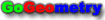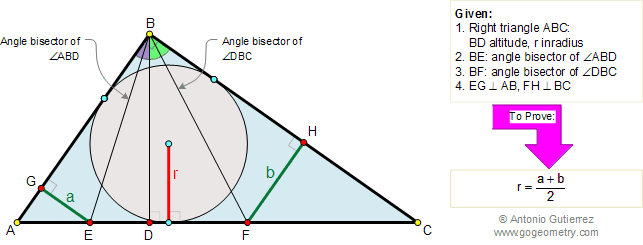Problem 220. Right Triangle, Altitude, Angle Bisector, Distance, Arithmetic Mean
 In a right triangle ABC, BD is the altitude to the hypotenuse AC and r is the inradius. BE and BF are the angle bisectors of angles ABD and DBC. Prove that r is the arithmetic mean of the measures of the perpendiculars from E and F to AB and BC respectively. View or post a solution.Home | Geometry | Altitude | Search | Problems | 211-220 | Right triangle, Altitude and Incircle | Email | View or post a solution